Courses

# Short Answer Type Questions(Part- 2)- Coordinate Geometry Class 10 Notes | EduRev

## Class 10 : Short Answer Type Questions(Part- 2)- Coordinate Geometry Class 10 Notes | EduRev

The document Short Answer Type Questions(Part- 2)- Coordinate Geometry Class 10 Notes | EduRev is a part of the Class 10 Course Class 10 Mathematics by VP Classes.
All you need of Class 10 at this link: Class 10

Q21. Show that the triangle PQR formed by the points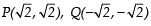and is an equilateral triangle.

OR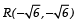Name the type of triangle PQR formed by the points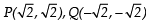and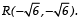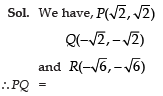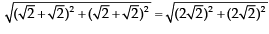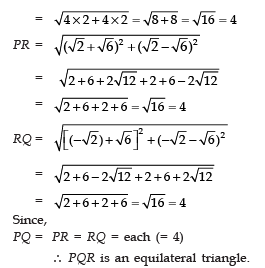Q22. The line joining the points (2, − 1) and (5, − 6) is bisected at P. If P lies on the line 2x + 4y + k = 0, find the value of k.

Sol. We have A (2, − 1) and B (5, − 6).
∵ P is the mid point of AB,
∴ Coordinates of P are: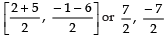Since P lies on the line 2x + 4y + k = 0
∴ We have: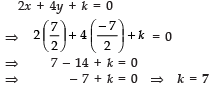Q23. Find the point on y-axis which is equidistant from the points (5, − 2) and (− 3, 2).

Sol. ∵ Let P is on the y-axis
∴ Coordinates of P are: (0, y)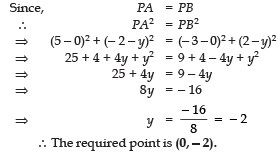Q24. The line joining the points (2, 1) and (5, − 8) is trisected at the points P and Q. If point P lies on the line 2x − y + k = 0, find the value of k.

Sol.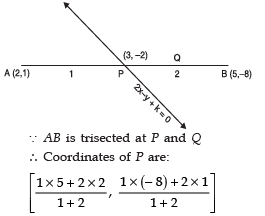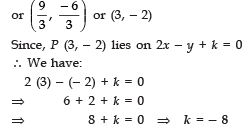Q25. If P (x, y) is any point on the line joining the points A (a, 0) and B (0, b), then show that: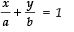Sol. ä P lies on the line joining A and B.
∴ A, B and P are collinear.
⇒ The area of a Δ formed by A (a, 0), B (0, b) and P (x, y) is zero.
∴ We have: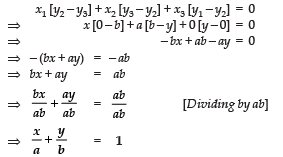Q. 26. Find the point on x-axis which is equidistant from the points (2, − 5) and (− 2, 9).

Sol. ∵ The required point ‘P’ is on x-axis.
∴ Coordinates of P are (x, 0).
∴ We have

AP = PB
⇒ AP2 = PB2
⇒ (2 − x)2 + (− 5 + 0)2 =(− 2 − x)2 + (9 − 0)2
⇒ 4 − 4x + x2 + 25 = 4 + 4x + x2 + 81
⇒ 4x + 25 = 4x + 81
⇒ − 8x = 56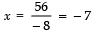∴ The required point is (−7, 0).

Q27. The line segment joining the points P (3, 3) and Q (6, − 6) is trisected at the points A and B such that A is nearer to P. It also lies on the line given by 2x + y + k = 0. Find the value of k.

Sol. ∵ PQ is trisected by A such that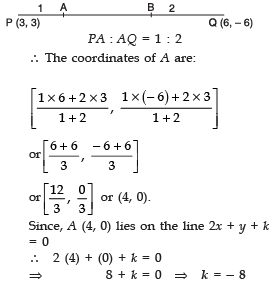Q28. Find the ratio in which the points (2, 4) divides the line segment joining the points A (− 2, 2) and B (3, 7). Also find the value of y.

Sol. Let P (2, y) divides the join of A (− 2, 2) and B (3, 7) in the ratio k:1
∴ Coordinates of P are: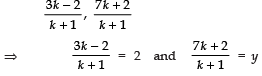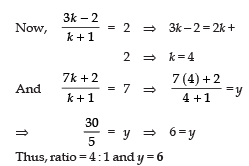Q29. Find the area of the quadrilateral ABCD whose vertices are: A (− 4, − 2), B (− 3, − 5), C (3, − 2) and D (2, 3)

Sol. Area of (Δ ABC)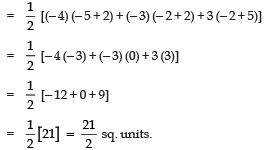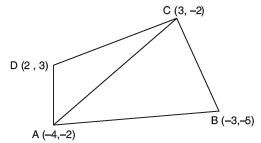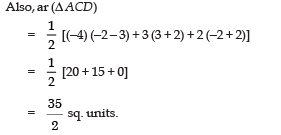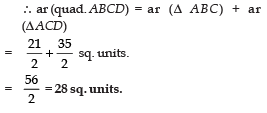Q30. Find the ratio in which the point (x, 2) divides the line segment joining the points (− 3, − 4) and (3, 5). Also find the value of x.

Sol. Let the required ratio = k : 1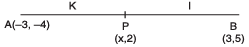∴ Coordinates of the point P are: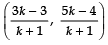But the coordinates of P are (x, 2)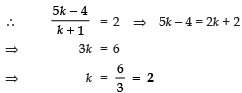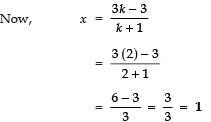Q31. If P (9a – 2, –b) divides the line segment joining A (3a + 1, −3 ) and B (8a, 5) in the ratio 3 : 1, find the values of a and b.

Sol. ∵ P divides AB in the ratio 3 : 1
∴ Using the section formula, we have: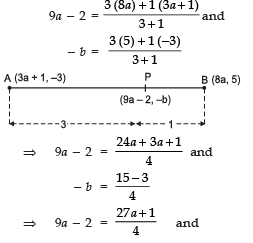− b = 3 or b = −3
⇒ 36a − 8= 27a + 1 and b= −3
⇒ 9a = 9 and b = −3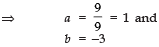Thus, the required value of a = 1 and b = −3

Q32. Find the area of the triangle formed by joining the mid-points of the sides of triangle whose vertices are (0, − 1), (2, 1), and (0, 3).

Sol. We have the vertices of the given triangle as A (0, − 1), B (2, 1) and C (0, 3). Let D, E and F be the mid-points of AB, BC and AC.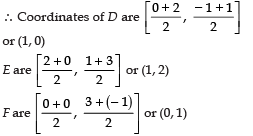∴ Coordinates of the vertices of Δ DEF are (1, 0), (1, 2) and (0, 1).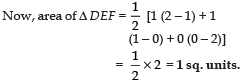Q33. Find the area of the Δ ABC with A(1, –4), and the mid-point of sides through A being (2, –1) and (0, –1).

Sol. Let the coordinates of B and C are (a, b) and (x, y) respectively.
Sides through A are AB and AC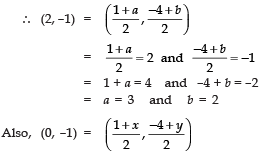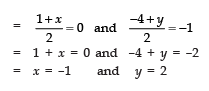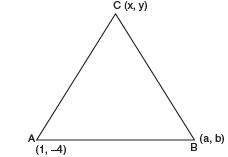Thus, the co-ordinates of the vertices of ΔABC are: A(1, –4), B(3, 2) and C(–1, 2)
∴ Area of ΔABC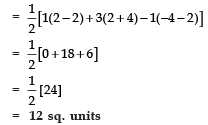Q34. Find the ratio in which the point (x, − 1) divides the line segment joining the points (− 3, 5) and (2, − 5). Also find the value of x.

Sol. Let the required ratio is k : 1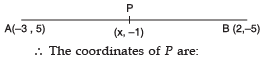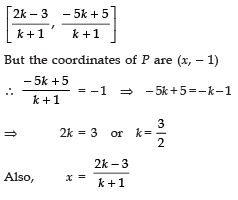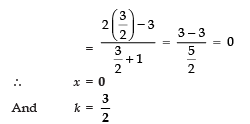Q35. Find the co-ordinates of the points which divide the line segment joining A(2, −3) and B(−4, −6) into three equal parts.

Sol. Let the required points are P(x1, y1) and Q(x2, y2)
∴ Using section formula, we have: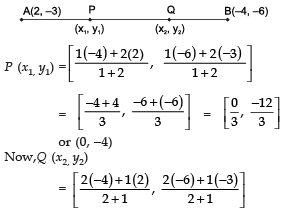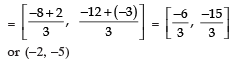Thus, the coordinates of the required points are (0, −4) and (−2, 5)

Q36. If the mid-point of the line segment joining the point A(3, 4) and B(k, 6) is P(x, y) and x + y – 10 = 0, then find the value of k.

Sol. ∵ Mid point of the line segment joining A(3, 4) and B(k, 6)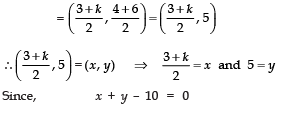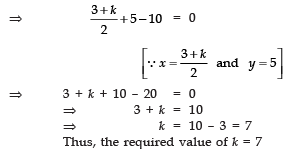Q37. Point P, Q, R and S divide the line segment joining the points A (1, 2) and B (6, 7) in 5 equal parts. Find the co-ordinates of the points P, Q and R.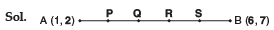∴  P, Q, R and S, divide AB into five      equal parts.
∴ AP = PQ = QR = RS = SB
Now, P divides AB in the ratio 1 : 4
Let, the co-ordinates of P be x and y.
∴ Using the section formula i.e.,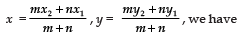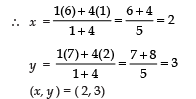Next, Q divides AB in the ratio 2 : 3
∴ Co-ordinates of Q are :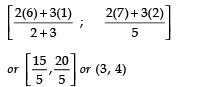Now, R divides AB in the ratio 3 : 2
⇒  Co-ordinates of R are :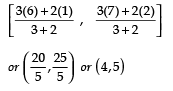The co-ordinates of P, Q  and R are respectively:(2, 3), (3, 4) and (4, 5).

Offer running on EduRev: Apply code STAYHOME200 to get INR 200 off on our premium plan EduRev Infinity!

132 docs

,

,

,

,

,

,

,

,

,

,

,

,

,

,

,

,

,

,

,

,

,

;